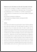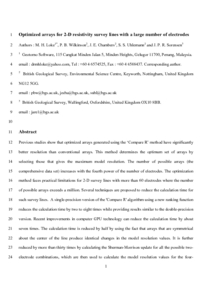nerc.ac.uk

Optimized arrays for 2-D resistivity survey lines with a large number of electrodes

Loke, M.H.; Wilkinson, P.B.; Chambers, J.E.; Uhlemann, S.S.; Sorensen, J.P.R.. 2015 Optimized arrays for 2-D resistivity survey lines with a large number of electrodes. Journal of Applied Geophysics, 112. 136-146. https://doi.org/10.1016/j.jappgeo.2014.11.011Preview
Text
Loke_et_al_2014_submitted.pdf - Accepted Version

Abstract/Summary

Previous studies show that optimized arrays generated using the ‘Compare R’ method have significantly better resolution than conventional arrays. This method determines the optimum set of arrays by selecting those that give the maximum model resolution. The number of possible arrays (the comprehensive data set) increases with the fourth power of the number of electrodes. The optimization method faces practical limitations for 2-D survey lines with more than 60 electrodes where the number of possible arrays exceeds a million. Several techniques are proposed to reduce the calculation time for such survey lines. A single-precision version of the ‘Compare R’ algorithm using a new ranking function reduces the calculation time by two to eight times while providing results similar to the double-precision version. Recent improvements in computer GPU technology can reduce the calculation time by about seven times. The calculation time is reduced by half by using the fact that arrays that are symmetrical about the center of the line produce identical changes in the model resolution values. It is further reduced by more than thirty times by calculating the Sherman–Morrison update for all the possible two-electrode combinations, which are then used to calculate the model resolution values for the four-electrode arrays. The calculation time is reduced by more then ten times by using a subset of the comprehensive data set consisting of only symmetrical arrays. Tests with a synthetic model and field data set show that optimized arrays derived from this subset produce inversion models with differences of less than 10% from those derived using the full comprehensive data set. The optimized data sets produced models that are more accurate than the Wenner–Schlumberger array data sets in all the tests.

Item Type: Publication - Article https://doi.org/10.1016/j.jappgeo.2014.11.011 0926-9851 GroundwaterBGS, groundwater, groundwater monitoring, Surface water interaction 12 Mar 2015 16:29 +0 (UTC) http://nora.nerc.ac.uk/id/eprint/510080View Item